×#### Thank you for registering.

One of our academic counsellors will contact you within 1 working day.

Click to Chat

1800-1023-196

+91-120-4616500

CART 0

• 0

MY CART (5)

Use Coupon: CART20 and get 20% off on all online Study Material

ITEM
DETAILS
MRP
DISCOUNT
FINAL PRICE
Total Price: Rs.

There are no items in this cart.
Continue Shopping
```
Consider four objects: (A), a solid sphere; (B), a spherical shell; (C), a solid disk; and (D), a metal hoop. All have the same mass and radius; all can be placed at the same point on the same inclined plane where they will roll without slipping to the bottom. The answer to the following questions might also be (E), all are the same. (a) Which object has the largest rotational inertia about its axis of symmetry? (b) If released from rest, which object will experience the largest net torque? (c) If released from rest, which object will experience the largest linear acceleration? (d) If allowed to roll down the incline, which object will have the largest speed at the bottom of the incline? (e) If allowed to roll down the incline, which object will reach the bottom of the incline in the shortest time?
Consider four objects: (A), a solid sphere; (B), a spherical shell; (C), a solid disk; and (D), a metal hoop. All have the same mass and radius; all can be placed at the same point on the same inclined plane where they will roll without slipping to the bottom. The answer to the following questions might also be (E), all are the same.(a) Which object has the largest rotational inertia about its axis of symmetry?(b) If released from rest, which object will experience the largest net torque?(c) If released from rest, which object will experience the largest linear acceleration?(d) If allowed to roll down the incline, which object will havethe largest speed at the bottom of the incline?(e) If allowed to roll down the incline, which object will reach the bottom of the incline in the shortest time?

```
5 years ago

```							(a)The correct option is (D) metal hoop.The rotational inertia of the solid sphere of is given as: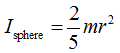Here, m is the mass of the sphere, and r is its radius.The rotational inertia of spherical shell about the diameter is given as: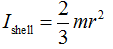Here, m is the mass of the sphere, and r is its radius.The rotational inertia of solid disk about the cylindrical axis is given as: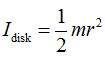Here, m is the mass of the disk, and r is its radius.The rotational inertia of metal hoop about the cylindrical axis is given as:(b)The correct option is (E).The torque experienced by the sphere is due to the presence of friction force fk given as:Therefore (E) is the correct option.(c)The correct option is (A) solid sphere.The linear acceleration of the object can be calculated using relation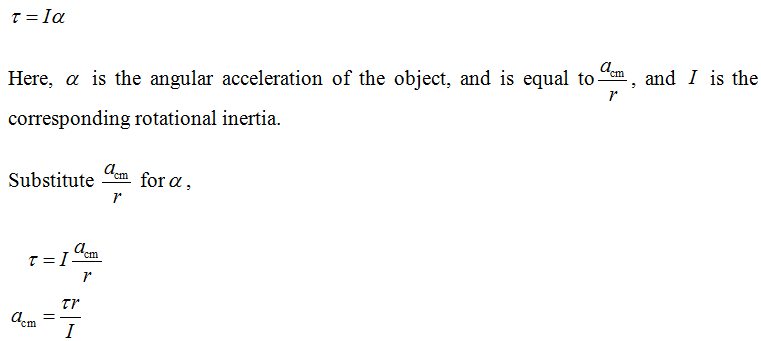From part (b), it has been concluded that the torque experienced by all the objects is the same, and since all objects have the same radius, one can deduce from equation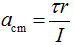that the linear acceleration of the object depends inversely on the rotational inertia of the object.Therefore, the object with minimum rotational inertia will have the largest linear acceleration.On comparing, calculated in part (a), one can conclude that rotational inertia of solid sphere is smallest amongst all. Therefore, the solid sphere will experience largest linear acceleration and (A) is the correct option.(d)The correct option is (A) solid sphere.Assume that the distance travelled by each object on inclined plane is d , and their initial velocity is vi . Given that each object is released from rest, one can ignore the term vi in the equation of motion.Using third equation of motion, one can write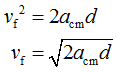Here, vf is the final velocity of the sphere, when it reaches the bottom of the inclined plane.It can be seen from equation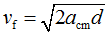, that the final speed of the object depends directly on the root of the linear acceleration ( being same for all objects). Therefore, the object moving with largest linear acceleration will have the largest linear speed at the bottom of the inclined plane.From part (c), one has that the linear acceleration of solid sphere is largest. Therefore, the solid sphere will have the largest speed at the bottom of the inclined plane. Thus, (A) is the correct option and the rest are ruled out.(e)The correct option is (A) solid sphere.The time taken to reach the bottom is given as:It can be seen from the equation above that the object will largest linear acceleration will reach down the inclined plane in shortest time. From part (c), it is clear that the largest linear acceleration is experienced by the solid sphere, and therefore it will reach down the inclined plane in shortest time.Thus (A) is the correct option and the rest are ruled out.
```
5 years ago
Think You Can Provide A Better Answer ?

## Other Related Questions on Mechanics

View all Questions »### Course Features

• 101 Video Lectures
• Revision Notes
• Previous Year Papers
• Mind Map
• Study Planner
• NCERT Solutions
• Discussion Forum
• Test paper with Video Solution### Course Features

• 110 Video Lectures
• Revision Notes
• Test paper with Video Solution
• Mind Map
• Study Planner
• NCERT Solutions
• Discussion Forum
• Previous Year Exam Questions